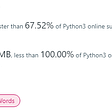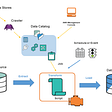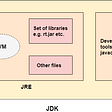# Median of Two Sorted Arrays — Day 36(Python)

Today’s question is one of Leetcode’s “Hard” tagged questions. It is a favorite among tech interviewers. Before we look into today’s problem, let us understand what a median is.

Median: The middle number; found by ordering all data points and picking out the one in the middle (or if there are two middle numbers, taking the mean of those two numbers).

Example: The median of 4, 1, and 7 is 4 because when the numbers are put in order (1, 4, 7), the number 4 is in the middle.

4. Median of Two Sorted Arrays

Given two sorted arrays `nums1` and `nums2` of size `m` and `n` respectively, return the median of the two sorted arrays.

Follow up: The overall run time complexity should be `O(log (m+n))`.

Example 1:

`Input: nums1 = [1,3], nums2 = Output: 2.00000Explanation: merged array = [1,2,3] and median is 2.`

Example 2:

`Input: nums1 = [1,2], nums2 = [3,4]Output: 2.50000Explanation: merged array = [1,2,3,4] and median is (2 + 3) / 2 = 2.5.`

Example 3:

`Input: nums1 = [0,0], nums2 = [0,0]Output: 0.00000`

Example 4:

`Input: nums1 = [], nums2 = Output: 1.00000`

Example 5:

`Input: nums1 = , nums2 = []Output: 2.00000`

Constraints:

• `nums1.length == m`
• `nums2.length == n`
• `0 <= m <= 1000`
• `0 <= n <= 1000`
• `1 <= m + n <= 2000`
• `-106 <= nums1[i], nums2[i] <= 106`

One of the easiest ways to solve this problem is by creating a list with numbers from both the list and then finding the median.

Initialize an empty list that stores the values in ascending order.
Since the given input lists are sorted, we would have to compare just the first elements in both the input list. Repeat the process until both the list are empty.

Once we have a complete list, we need to find the median for the resultant array.

If the length of the resultant list is odd, the median is the middle element in the list.

If the the length of the resultant list is even, the median is the mean of two middle element in the list.

Let us look at the code snippet.

`class MedianFinder:    def findMedianSortedArrays(self, nums1: List[int], nums2: List[int]) -> float:        output_list = []        while(nums1 and nums2):            if nums1 <= nums2:                output_list.append(nums1.pop(0))            else:                output_list.append(nums2.pop(0))        while nums1:            output_list.append(nums1.pop(0))        while nums2:            output_list.append(nums2.pop(0))        mid = len(output_list)//2        if len(output_list)%2 != 0:            return(output_list[mid])        return(float(output_list[mid] + output_list[mid-1])/2)`

Complexity analysis.

Time Complexity

Since we are building a new array that includes elements from both the array, the time complexity for the above solution is O(M+N), where M is the size of array1 and N is the size of array2.

Space Complexity

Since we are building a new array that includes elements from both the array, the space complexity for the above solution is O(M+N), where M is the size of array1 and N is the size of array2.

Can we try to reduce time/space complexity? Do we have to create a new array to find the median? The given list is sorted, can we use this information to find out the median?

When we are trying to find the median of a list, everything to the left of this number, which is the median, should be smaller, while everything to the right of this number should be bigger. If we use the same logic concerning two lists, we need to find a partition in such a way that the number from both the list to the left of the partition is smaller, while the number to the right of the partition is bigger. Hence the basic idea here is to find a proper partition. To find a partition, we can use binary search since we have the lists sorted. After we find partitions, we will have four numbers that we need to consider.

We need to ensure that we find a partition in such a way that,

X1 < Y2 and Y1 < X2.

Until we find this partition, we need to keep performing our binary search.

Let us look into the algorithm.

1. List 1 should be smaller than List 2 in size. In case the condition is false, exchange List 1 and List 2.
2. We would require two-variable “start” and “end”, which keeps track of the start and end position of our list 1(smaller list).
3. Variable “X” and “Y” will signify the length of list 1 and list 2, respectively.
4. Run the loop until “start” is less than or equal to “end”.
5. We have two variables that determine the partition in both the list. Let us call it “PartitionX” and “PartitionY”. The formula for “PartitionX” is mid of start and end. The formula for “PartitionY” is ((length of list 1 + length of list 2) / 2)-PartitionX.
6. If we find the correct partition, i.e. (X1 <= Y2) and (Y1 <= X2), we move on to find the median. If the sum of the length of both lists is even, then the formula to find median is ((max(X1, Y1) + min(X2, Y2))/2). If the sum is odd, then the median is max(X1, Y1).
7. If we have not found the correct partition, i.e. if Y1 > X2, then, the start is equal to PartitionX + 1.
8. If we have not found the correct partition, i.e. if X1 > Y2, then, the end is equal to PartitionX — 1.

Few things to keep in mind. If any of the Partition is equal to 0, then the number to the left of Partition is equal to float(‘-inf’). If any of the Partition is equal to the end of the list, then the number to the right of Partition is equal to float(‘inf’).

Let us look into the code snippet.

`class MedianFinder:    def findMedianSortedArrays(self, nums1: List[int], nums2: List[int]) -> float:        if(len(nums1) > len(nums2)):            return self.findMedianSortedArrays(nums2, nums1)        array_1 = nums1        array_2 = nums2        start = 0        end = len(array_1)        X = len(array_1)        Y = len(array_2)        while(start <= end):                        partitionX = int((start + end )/2)            partitionY = int((X + Y + 1 )/2 - partitionX)            #Edge case when there is nothing on the left side, then we assign x1 to infinity            if(partitionX == 0):                X1 = float('-inf')            else:                X1 = array_1[partitionX - 1]                            if(partitionX == len(array_1) ):                X2 = float('inf')            else:                X2 = array_1[partitionX]                            if(partitionY == 0):                Y1 = float('-inf')            else:                Y1 = array_2[partitionY - 1]                            if(partitionY == len(array_2) ):                Y2 = float('inf')            else:                Y2 = array_2[partitionY]            if((X1 <= Y2) and (Y1 <= X2)):                                # We have found correct partitions                                #Check if the sum of length of both is odd or even                                if( (X+Y) % 2 == 0):                                        median = ((max(X1,Y1) + min(X2, Y2))/2)                    return median                else:                                        median = max(X1,Y1)                    return median                            elif(Y1 > X2):                start = partitionX + 1            else:                end = partitionX - 1`

Complexity analysis.

Time Complexity

We are using a binary search to find the median. We know the time complexity of a binary search is O(logN). In our case, the time complexity is O(log(M+N)).

Space Complexity

We are not using any extra data structure to store any intermediate results, and hence space complexity is O(1).

I referred to the following video to find a solution to the given problem.

I would love to hear your feedback about my posts. Do let me know if you have any comments or feedback.

--

--

--

## More from Annamariya Tharayil

Software Engineer. Find me @ www.linkedin.com/in/annamariya-jt

Love podcasts or audiobooks? Learn on the go with our new app.

## Creating a Chatbot for Healthcare in React Native using Dialogflow -## [LeetCode][python3]0012. Integer to Roman## PMP Exam is changing in December, 2019 — pmwares## Multiple Return Values: Golang## AWS Athena## How to effectively choose a tech vendor partner## Annamariya Tharayil

Software Engineer. Find me @ www.linkedin.com/in/annamariya-jt

## JVM vs JDK vs JRE